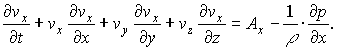# Euler´s equation for inviscid flow

Hello,
i would like to simulate an ideal gas flow in a cube...the cube consists of small cubes lined next to each other..after warming up one small cube i should describe how is the heat spreading to the rest of the smaller cubes...using eulers equations for inviscid fluid motion 3D..
using the equation of state I count the mass of molecules inside one small cube,a=1m
V=1m3
P=100KPa
T=293.15K
r=8.314
n=PV/rT
n=41.0299mol
------------------------
i warm up one small cube to 293.25K,so the pressure will rise

now according to the equation for axes xit should be something like
Vx_new-Vx+(Vx-V(x-1))*Vx=-(Px-P(x-1))/rho
where
Vx_new-Vx=local acceleration
(Vx-V(x-1))*Vx=konvective acceleration
the rest can be neglected cause it is laminar flow
----------------------------
as far as i know the molecules in the cube with higher pressure should move to cubes with lower pressure
------------------------------
the problem is that using this formula the molecules spread also from cubes with lower pressure to cube with higher pressure which is wrong..and i don t know how to twist the formula to prevent that..
-------------------------
so please if u have some experiences with modeling gas flow using there equations or if u see a mistake in my rewritten formula please let me know..
--------------------------

I think you shouldn't omit the last two summands in the formula for the total derivative of velocity. Also you didn't write how you adjusted the pressure after each time step: you should use continuity equation both for mass and energy (to determine the amount and temperature of the gass in each cell, which are needed to calculate the pressure).
Movement of the molecules towards higher pressure is in fact physically possible, since the pressure only determines the acceleration of the molecules and not the speed: if they have enough speed, then they can move towards higher pressure for some time.

Last edited:

I was told that by laminar flow the last two summands can be ommited
the general idea is>
By computing Vx(new) i get the speed of the molecules
Then i take each cube and move it to the right direction
The source cube should donate molecules(warmth) to other cubes
T=T1*n1+T2*n2.../n1+n2...
And then i compute pressure again with new "T" and "n" and do the same in rounds
the heat should spread all over the cube..
The process is described on the picture..I used 2D model

View attachment cube.bmp

I don't understand your calculation of donated molecules. Their amount should increase lineary with time step dt, while it seems to be proportional to dt^2 in your formula. I understood that you took 1 s for your time step. That is probably too much (at least while your typical velocity*dt gives the same order of magnitude as box size a): numerical solving of diferential equations works well only for very small time steps (that cause small relative changes of parameters in each step).
I'm still not convinced about the two omited summands in the total derivatives of velocity: maybe you were supposed to define coordinate system with x axes parallel to velocity (at each box)? (then those parts are of course zero)

Ok,so i can change the time step to 0.01s for example,can you tell me what your suggestion for computing donation of molecules and heat is?..
i thought that the geometrical soulution is good enough
for 3D:
amouth of molecules in cube/total volume of the cube=
amount of molecules for next cube/volume of the molecules for next cube

n/V=n1/V1
n1=n*V1/V

..the thing is that whether i omit the last two summands or not i still think there is something wrong with that..one thing can be the time step,but it is not the only..

The expression for molecules passing from cube [0,0] to [0,1]

nd=n[0,0]*(x1*y1)/a^2 should be replaced by

nd=n[0,0]*(x1*(a-y1))/a^2

If you chose small time step dt then y1<<a and the expression becomes aproximately:

nd=n[0,0]*x1/a

Of course you should sum contributions from all neighbors of each cube in each time step (except diagonal neighbours: their contribution is negligible if you chose small dt, since it is proportional to dt^2).

re

thank you so much i see your point..
..i tried to compute something on paper for x-axis only,and that seems to be the biggest problem
take a look
View attachment x_axis.bmp
i don t like the flow..i don t think it is correct..